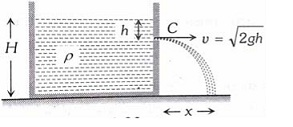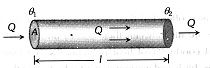Q

what is the difference between the two ?

H Himanshu Meshram

The prefix poly means many. So the word polynomial refers to one or more than one term in an expression. The relationship between these terms may be sums or differences. You call expression with a single term a monomial, an expression with two terms is a binomial, and expression with three terms is a trinomial. Here we are only discussing Binomial along with their exponents.

Why we study iota?

H Himanshu Meshram
In general, numbers are of two types imaginary number and a real number A real number can be studied easily but an imaginary number shows a different type of property which we will study in this topic by using iota.

Interference pattern is observed at ‘P’ due to superimposition of two rays coming out
from a source ‘S’ as shown in the figure.The value of ‘’ for which maxima is
obtained at ‘P’ is : (R is perfect reflecting surface) :• Option 1)• Option 2)• Option 3)• Option 4)V Vakul

As we have learned

Lloyd's mirror Experiment -

- wherein

minima

maxima

For maxima at POr

or

Option 1)Option 2)Option 3)Option 4)The circuit has two oppositely connect ideal diodes in parallel. What is the current following in the circuit?• Option 1)

1.33 A

• Option 2)

1.71 A

• Option 3)

2.00 A

• Option 4)

2.31 A.

A Avinash

As we have learnt,

P -N junction as diode -

It is a one way device. It offers a low resistance when forward biased and high resistance when reverse biased.

- wherein

R = 0, Forward

ReverseIn the figure D, is reverse biased, hence no current will flow through D.

Option 1)

1.33 A

Option 2)

1.71 A

Option 3)

2.00 A

Option 4)

2.31 A.

In a full wave rectifier circuit operating from 50 Hz mains frequency, the fundamental frequency in the ripple would be

• Option 1)

100 Hz

• Option 2)

70.7 Hz

• Option 3)

50 Hz

• Option 4)

25 Hz

A Avinash

As we have learnt,

Full wave rectifier -

It is type of rectification in which output is obtained for both the halves of a.c.

- wherein

Frequency of output is double to that of input

In full wave rectifier

Input                                                                                   OutputTime period becomes half

Frequency gets doubled

Frequency is 100 Hz

Option 1)

100 Hz

Option 2)

70.7 Hz

Option 3)

50 Hz

Option 4)

25 Hz

If in a   junction diode, a square input signal of 10 V is applied as shownThen the output signal across will be

• Option 1)• Option 2)• Option 3)• Option 4)A Avinash

As we have learnt,

P -N junction as diode -

It is a one way device. It offers a low resistance when forward biased and high resistance when reverse biased.

- wherein

R = 0, Forward

ReverseWhen input is -5V, then diode is reverse biased and hence output is zero

When input is +5V, then output is also +5V

Option 1)Option 2)Option 3)Option 4)The machine as shown has 2 rods of length 1 m connected by a pivot at the top.  The end of one rod is connected to the floor by a stationary pivot and the end of the other rod has a roller that rolls along the floor in a slot.  As the roller goes back and forth, a 2 kg weight moves up and down.  If the roller is moving towards right at a constant speed, the weight moves up with a :• Option 1)

constant speed

• Option 2)

decreasing speed

• Option 3)

increasing speed

• Option 4)

speed which isth of that of the roller when the weight is 0.4 m above the ground

S subamFrom

dx /dt = constant

As with motion of roller , x decreases

dy/dt decreases

weight will move with decreasing speed

Option 1)

constant speed

Option 2)

decreasing speed

Option 3)

increasing speed

Option 4)

speed which isth of that of the roller when the weight is 0.4 m above the ground

A line drawn through the point P(4, 7) cuts the circle x2+y2=9 at the points A and B. Then PA⋅PB is equal to :

• Option 1)

53

• Option 2)

56

• Option 3)

74

• Option 4)

65

S satyajeet

As we learnt

Length of a tangent -

- wherein

Length of tangent from a external point   to circleSince we know,

Option 1)

53

Option 2)

56

Option 3)

74

Option 4)

65

A spring of unstretched length l has a mass m with one end fixed to a rigid support. Assuming spring to be made of a uniform wire, the kinetic energy possessed by it if its free end is pulled with uniform velocityis :

• Option 1)• Option 2)• Option 3)• Option 4)S SudhirSol

As we have learned

Kinetic energy -

- wherein

Kinetic Energy is always positive.fixed end is at rest and free end is moving with speed v

Velocity at distance x from free end

Kinetic energy of mass dm at x = x is K.E =

Total kinetic energy =

Option 1)Option 2)Option 3)Option 4)Two soap bubbles coalesce to form a single bubble. If V is the subsequent change in volume of contained air and S the change  in total surface area, T is the surface  tension and P atmospheric pressure, which  of the following relation is correct ?

• Option 1)• Option 2)• Option 3)• Option 4)S SudhirSol

As we have learned

Change in Pressure of bubble in air -

- wherein

T- TemperatureOption 1)Option 2)Option 3)Option 4)A tank with a small hole at the bottom has been filled with water and kerosene (specific gravity 0.8). The height of water is 3 m and that of kerosene 2 m. When the hole is opened the velocity of fluid coming out from it is nearly : (take g=10 ms-2 and density of water =103 kg m-3)

• Option 1)

10.7 ms-1

• Option 2)

9.6 ms-1

• Option 3)

8.5 ms-1

• Option 4)

7.6 ms-1

S SudhirSol

As we have learned

Torricelli's Theorem / Velocity of Efflux -

In fluid dynamics relating the speed of fluid flowing out of an orifice.

- whereinAccording to torcellli theorem

velocity of efflux =

Left density of water is

Density of kerosene = 0. 8

Apply bernoulli between A and B

Option 1)

10.7 ms-1

Option 2)

9.6 ms-1

Option 3)

8.5 ms-1

Option 4)

7.6 ms-1

A cylindrical vessel of cross-section A contains water to a height h.There is a hole in the bottom of radius 'a'.The time in which it will be emptied is :

• Option 1)• Option 2)• Option 3)• Option 4)S SudhirSol

As we have learned

Torricelli's Theorem / Velocity of Efflux -

In fluid dynamics relating the speed of fluid flowing out of an orifice.

- whereinVelocity of eflux of water =

Option 1)Option 2)Option 3)Option 4)The figure shows a system of two concentric spheres of radii and kept at temperatures respectively. The radial rate of flow of heat in a substance between the two concentric spheres is proportional to

• Option 1)

• Option 2)

• Option 3)

• Option 4)

S SudhirSol

As we have learned

Thermal Conductivity -

K = thermal conductivity

- whereinRate of flow of heat

We have a spherical shell of radius r and thickness dx

Option 1)

Option 2)

Option 3)

Option 4)

Water is flowing at a speed of 1.5 ms-1 through a horizontal tube of cross-sectional area 10-2 m2 and you are trying to stop the flow by your palm. Assuming that the water stops immediately after hitting the palm, the minimum force that you must exert should be (density of water=103 kgm-3).

• Option 1)

15 N

• Option 2)

22.5 N

• Option 3)

33.7

• Option 4)

45 N

S SudhirSol

As we have learned

Equation of Continuity -

Mass of the liquid entering per second at A = mass of the liquid leaving per second at B.

a1 v1 = a2 v2

- wherein

a1  and abe the area of cross section.Let us say speed of water is v and area of cross section is A

In one second mass of water that flows is

F = momentum change per second

Option 1)

15 N

Option 2)

22.5 N

Option 3)

33.7

Option 4)

45 N

An open glass tube is immersed in mercury in such a way that a length of 8 cm extends above the mercury level. The open end of the tube is then closed and sealed and the tube is raised vertically up by additional 46 cm. What will be length of the air column above mercury in the tube now ?

(Atmospheric pressure =76 cm of Hg)

• Option 1)

16 cm

• Option 2)

22 cm

• Option 3)

38 cm

• Option 4)

6 cm

S SudhirSol

As We have learned

Absolute Pressure -

- whereinFor air trapped in tube T = constant

Option 1)

16 cm

Option 2)

22 cm

Option 3)

38 cm

Option 4)

6 cm

Exams
Articles
Questions